# NON-CATEGORICAL VARIABLESChapter I - A Survey of Bayesian Data Mining Data Mining: Opportunities and Challenges by John Wang (ed) Idea Group Publishing 2003Brought to you by Team-Fly

We will now consider data matrices made up from ordinal and real valued data, and then matrices consisting of ordinal, real, and categorical data. The standard choice for a real valued data model is the univariate or multivariate Gaussian or normal distribution. It has nice theoretical properties manifesting themselves in such forms as the central limit theorem, the least squares method, principal components, etc. It is possible to formulate the theory of the model choice section using inverse Wishart distributions as conjugate priors for multivariate normal distributions, but this is leads to fairly complex formulas and is seldom implemented (Bernardo & Smith, 1994). The normal distribution is also unsatisfactory for many data sets occurring in practice, because of its thin tail and because many real life distributions deviate terribly from it. Several approaches to solve this problem are available. One is to consider a variable as being obtained by mixing several normal distributions. Another is to disregard the distribution over the real line, and considering the variable as just being made up of an ordered set of values. A quite useful and robust method is to discretize the variables. This is equivalent to assuming that their probability distribution functions are piecewise constant. Discretized variables can be treated as categorical variables by the methods described above. The methods waste some information, but are quite simple and robust. Typically, the granularity of the discretization is chosen so that a reasonably large number of observations fall in each level. A compromise between discretization and use of continuous distributions is analyses of the rankings of the variables occurring in data tables. When considering the association between a categorical and a continuous variable one would thus investigate the ranks of the continuous variable, which are uniformly distributed over their range for every category if there is no association. Using a model where the ranks are non-uniformly distributed (e.g., with a linearly varying density), we can build the system of model comparisons of the model choice section. The difficulty is that the nuisance parameters cannot be analytically integrated out, so a numerical or MCMC quadrature procedure must be used.

A review of distributions used by statisticians and their parameter estimation methods is found in Chapter 11.

### Missing Values in Data Matrix

Data collected from experiments are seldom perfect. The problem of missing and erroneous data is a vast field in the statistics literature. First of all, there is a possibility that "missingness" of data values are significant for the analysis, in which case missingness should be modeled as an ordinary data value. Then the problem has been internalized, and the analysis can proceed as usual, with the important difference being that the missing values are not available for analysis. A more skeptical approach was developed by Ramoni and Sebastiani(1998), who consider an option to regard the missing values as adversaries (the conclusions on dependence structure would then be true, no matter what the missing values are but with lots of missing data, conclusions will become very weak). A third possibility is that missingness is known to have nothing to do with the objectives of the analysis data are missing completely at random. For example, in a medical application, if data is missing because of the bad condition of the patient, missingness is significant if the investigation is concerned with patients. But if data is missing because of unavailability of equipment, it is probably not - unless maybe if the investigation is related to hospital quality.

Assuming that data is missing completely at random, it is relatively easy to get an adequate analysis. It is not necessary to waste entire cases just because they have a missing item. Most of the analyses made refer only to a small number of columns, and these columns can be compared for all cases that have no missing data in these particular columns. In this way it is, for example, possible to make a graphical model for a data set even if every case has some missing item, since all computations of the graphical model choice section refer to a small number of columns. In this situation, it is even possible to impute the values missing, because the graphical model obtained shows which variables most influence the missing one. So every missing value for a variable can be predicted from values of the case for neighbors of the variable in the graph of the model. When this is done, one must always remember that the value is a guess. It can thus never be used to create a formal significance measure - that would be equivalent to using the same data twice, which is not permitted in formal inference. A comprehensive review of the missing data problem can be found in Chapter 7.

The method of imputing missing values has a nice formalization in the Expectation Maximization (EM) method. This method is used to create values for missing data items by using a parameterized statistical model of the data. In the first step, the non-missing data is used to create an approximation of the parameters. Then the missing data values are defined (given imputed values) to give highest probability to the imputed data matrix. We can then refine the parameter estimates by maximization of probability over parameters with the now imputed data, then over the missing data, etc., until convergence results. This method is recommended for use in many situations, despite the fact that it is not strictly Bayesian and it violates the principle of not creating significance from guessed (imputed) values. The most spectacular use of the EM algorithm is for automatic (unsupervised) classification in the AUTOCLASS model (see next subsection).

### Segmentation - Latent Variables

Segmentation and latent variable analysis aims at describing the data set as a collection of subsets, each having simpler descriptions than the full data matrix. The related technique of cluster analysis, although not described here, can also be given a Bayesian interpretation as an effort to describe a data set as a collection of clusters with small variation around the center of each cluster. Suppose data set D is partitioned into dc classes {D(i)}, and each of these has a high posterior probability p(D(i) Mi) wrt some model set Mi. Then we think that the classification is a good model for the data. However, some problems remain to consider. First, what is it that we compare the classification against, and second, how do we accomplish the partitioning of the data cases into classes? The first question is the simplest to answer: we compare a classification model against some other model, based on classification or not. The second is trickier, since the introduction of this section is somewhat misleading. The prior information for a model based on classification must have some information about classes, but it does not have an explicit division of the data into classes available. Indeed, if we were allowed to make this division into classes on our own, seeking the highest posterior class model probabilities, we would probably over-fit by using the same data twice once for class assignment and once for posterior model probability computation. The statistical model generating segmented data could be the following: a case is first assigned to a class by a discrete distribution obtained from a suitable uninformative Dirichlet distribution, and then its visible attributes are assigned by a class-dependent distribution. This model can be used to compute a probability of the data matrix, and then, via Bayes' rule, a Bayes factor relating the model with another one, e.g., one without classes or with a different number of classes. One can also have a variable number of classes and evaluate by finding the posterior distribution of the number of classes. The data probability is obtained by integrating, over the Dirichlet distribution, the sum over all assignments of cases to classes, of the assignment probability times the product of all resulting case probabilities according to the respective class model. Needless to say, this integration is feasible only for a handful of cases where the data is too meager to permit any kind of significant conclusion on the number of classes and their distributions. The most well-known procedures for automatic classification are built on expectation maximization. With this technique, a set of class parameters are refined by assigning cases to classes probabilistically, with the probability of each case membership determined by the likelihood vector for it in the current class parameters (Cheeseman & Stutz, 1995). After this likelihood computation, a number of cases are moved to new classes to which they belong with high likelihood. This procedure converges to a local maximum, where each case with highest likelihood belongs to its current class. But there are many local maxima, and the procedure must be repeated a number of times with different starting configurations.

### Basic Classification

We assume that rows are generated as a finite mixture with a uniform Dirichlet prior for the component (class) probabilities, and each mixture component has its rows generated independently, according to a discrete distribution also with a uniform Dirichet prior for each column and class. Assume that the number of classes C is given, the number of rows is n and the number of columns is K, and that there are dk different values that can occur in column k. For a given classification, the data probability can be computed; let ni(c,k) be the number of occurrences of value i in column k of rows belonging to class c. Let xi(c,k) be the probability of class c having the value i in column k. Let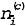be the number of occurences in class c of the row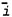, and n(c) the number of rows of class c. By equation (3) the probability of the class assignment depends only on the number of classes and the table size, Γ(n +1)Γ(C)/Γ(n + C). The probability of the data in class c is, if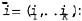: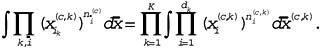The right side integral can be evaluated using the normalization constant of the Dirichlet distribution, giving the total data probability of a classification: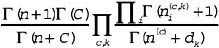The posterior class assignment distribution is obtained normalizing over all class assignments. This distribution is intractable, but can be approximated by searching for a number of local maxima and estimating the weight of the neighborhood of each maximum. Here the EM algorithm is competitive to MCMC calculations in many cases, because of the difficulty of tuning the proposal distribution of MCMC computations to avoid getting stuck in local minima. The procedure is to randomly assign a few cases to classes, estimate parameters xi(c,k), assign remaining cases to optimum classes, recomputing the distribution of each case over classes, reclassifying each case to optimum class, and repeating until convergence. After repeating this procedure for a while, one typically finds a single, most probable class assignment for each number of classes. The set of local optima so obtained can be used to guide a MCMC simulation giving more precise estimates of the probabilities of the classifications possible. But in practice, a set of high-probability classifications is normally a starting point for application specialists trying to give application meaning to the classes obtained.

 Brought to you by Team-Fly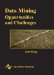Data Mining: Opportunities and Challenges
ISBN: 1591400511
EAN: 2147483647
Year: 2003
Pages: 194
Authors: John Wang